## CodeNewbie Community 🌱# 150 JavaScript Questions Part 1

In this post, we can provide 50 multiple-choice JavaScript questions and answers, with the help of this you can prepare your javascript interview easily.

If you like this post so we can provide the next 50 + 50 questions and answers in the next part.

## 150 JavaScript Questions Part 1

###### 1. What's the output?
``````function sayHi() {
console.log(name);
console.log(age);
var name = 'Lydia';
let age = 21;
}

sayHi();
``````
• A: `Lydia` and `undefined`
• B: `Lydia` and `ReferenceError`
• C: `ReferenceError` and `21`
• D: `undefined` and `ReferenceError`

Answer: D Within the function, we first declare the `name` variable with the `var` keyword. This means that the variable gets hoisted (memory space is set up during the creation phase) with the default value of `undefined`, until we actually get to the line where we define the variable. We haven't defined the variable yet on the line where we try to log the `name` variable, so it still holds the value of `undefined`. Variables with the `let` keyword (and `const`) are hoisted, but unlike `var`, don't get initialized. They are not accessible before the line we declare (initialize) them. This is called the "temporal dead zone". When we try to access the variables before they are declared, JavaScript throws a `ReferenceError`.

###### 2. What's the output?
``````for (var i = 0; i < 3; i++) {
setTimeout(() => console.log(i), 1);
}

for (let i = 0; i < 3; i++) {
setTimeout(() => console.log(i), 1);
}
``````
• A: `0 1 2` and `0 1 2`
• B: `0 1 2` and `3 3 3`
• C: `3 3 3` and `0 1 2`

Answer: C Because of the event queue in JavaScript, the `setTimeout` callback function is called _after_ the loop has been executed. Since the variable `i` in the first loop was declared using the `var` keyword, this value was global. During the loop, we incremented the value of `i` by `1` each time, using the unary operator `++`. By the time the `setTimeout` callback function was invoked, `i` was equal to `3` in the first example. In the second loop, the variable `i` was declared using the `let` keyword: variables declared with the `let` (and `const`) keyword are block-scoped (a block is anything between `{ }`). During each iteration, `i` will have a new value, and each value is scoped inside the loop.

###### 3. What's the output?
``````const shape = {
diameter() {
},
perimeter: () => 2 * Math.PI * this.radius,
};

console.log(shape.diameter());
console.log(shape.perimeter());
``````
• A: `20` and `62.83185307179586`
• B: `20` and `NaN`
• C: `20` and `63`
• D: `NaN` and `63`

Answer: B Note that the value of `diameter` is a regular function, whereas the value of `perimeter` is an arrow function. With arrow functions, the `this` keyword refers to its current surrounding scope, unlike regular functions! This means that when we call `perimeter`, it doesn't refer to the shape object, but to its surrounding scope (window for example). There is no value `radius` on that object, which returns `NaN`.

###### 4. What's the output?
``````+true;
!'Lydia';
``````
• A: `1` and `false`
• B: `false` and `NaN`
• C: `false` and `false`

Answer: A The unary plus tries to convert an operand to a number. `true` is `1`, and `false` is `0`. The string `'Lydia'` is a truthy value. What we're actually asking, is "is this truthy value falsy?". This returns `false`.

###### 5. Which one is true?
``````const bird = {
size: 'small',
};

const mouse = {
name: 'Mickey',
small: true,
};
``````
• A: `mouse.bird.size` is not valid
• B: `mouse[bird.size]` is not valid
• C: `mouse[bird["size"]]` is not valid
• D: All of them are valid

Answer: A In JavaScript, all object keys are strings (unless it's a Symbol). Even though we might not _type_ them as strings, they are always converted into strings under the hood JavaScript interprets (or unboxes) statements. When we use bracket notation, it sees the first opening bracket `[` and keeps going until it finds the closing bracket `]`. Only then, it will evaluate the statement. `mouse[bird.size]`: First it evaluates `bird.size`, which is `"small"`. `mouse["small"]` returns `true` However, with dot notation, this doesn't happen. `mouse` does not have a key called `bird`, which means that `mouse.bird` is `undefined`. Then, we ask for the `size` using dot notation: `mouse.bird.size`. Since `mouse.bird` is `undefined`, we're actually asking `undefined.size`. This isn't valid, and will throw an error similar to `Cannot read property "size" of undefined`.

###### 6. What's the output?
``````let c = { greeting: 'Hey!' };
let d;

d = c;
c.greeting = 'Hello';
console.log(d.greeting);
``````
• A: `Hello`
• B: `Hey!`
• C: `undefined`
• D: `ReferenceError`
• E: `TypeError`

Answer: A In JavaScript, all objects interact by _reference_ when setting them equal to each other. First, variable `c` holds a value to an object. Later, we assign `d` with the same reference that `c` has to the object.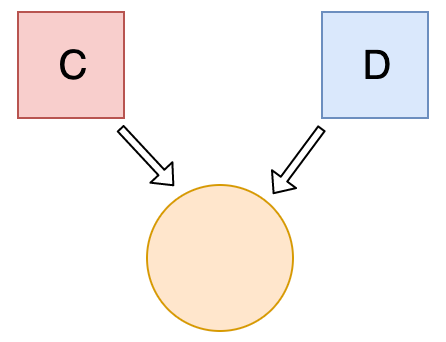When you change one object, you change all of them.

###### 7. What's the output?
``````let a = 3;
let b = new Number(3);
let c = 3;

console.log(a == b);
console.log(a === b);
console.log(b === c);
``````
• A: `true` `false` `true`
• B: `false` `false` `true`
• C: `true` `false` `false`
• D: `false` `true` `true`

Answer: C `new Number()` is a built-in function constructor. Although it looks like a number, it's not really a number: it has a bunch of extra features and is an object. When we use the `==` operator, it only checks whether it has the same _value_. They both have the value of `3`, so it returns `true`. However, when we use the `===` operator, both value _and_ type should be the same. It's not: `new Number()` is not a number, it's an **object**. Both return `false.`

###### 8. What's the output?
``````class Chameleon {
static colorChange(newColor) {
this.newColor = newColor;
return this.newColor;
}

constructor({ newColor = 'green' } = {}) {
this.newColor = newColor;
}
}

const freddie = new Chameleon({ newColor: 'purple' });
console.log(freddie.colorChange('orange'));
``````
• A: `orange`
• B: `purple`
• C: `green`
• D: `TypeError`

Answer: D The `colorChange` function is static. Static methods are designed to live only on the constructor in which they are created, and cannot be passed down to any children. Since `freddie` is a child, the function is not passed down, and not available on the `freddie` instance: a `TypeError` is thrown.

###### 9. What's the output?
``````let greeting;
greetign = {}; // Typo!
console.log(greetign);
``````
• A: `{}`
• B: `ReferenceError: greetign is not defined`
• C: `undefined`

Answer: A It logs the object, because we just created an empty object on the global object! When we mistyped `greeting` as `greetign`, the JS interpreter actually saw this as `global.greetign = {}` (or `window.greetign = {}` in a browser). In order to avoid this, we can use `"use strict"`. This makes sure that you have declared a variable before setting it equal to anything.

###### 10. What happens when we do this?
``````function bark() {
console.log('Woof!');
}

bark.animal = 'dog';
``````
• A: Nothing, this is totally fine!
• B: `SyntaxError`. You cannot add properties to a function this way.
• C: `"Woof"` gets logged.
• D: `ReferenceError`

Answer: A This is possible in JavaScript, because functions are objects! (Everything besides primitive types are objects) A function is a special type of object. The code you write yourself isn't the actual function. The function is an object with properties. This property is invocable.

###### 11. What's the output?
``````function Person(firstName, lastName) {
this.firstName = firstName;
this.lastName = lastName;
}

const member = new Person('Lydia', 'Hallie');
Person.getFullName = function() {
return `\${this.firstName} \${this.lastName}`;
};

console.log(member.getFullName());
``````
• A: `TypeError`
• B: `SyntaxError`
• C: `Lydia Hallie`
• D: `undefined` `undefined`

Answer: A In JavaScript, functions are objects, and therefore, the method `getFullName` gets added to the constructor function object itself. For that reason, we can call `Person.getFullName()`, but `member.getFullName` throws a `TypeError`. If you want a method to be available to all object instances, you have to add it to the prototype property: ```js Person.prototype.getFullName = function() { return `\${this.firstName} \${this.lastName}`; }; ```

###### 12. What's the output?
``````function Person(firstName, lastName) {
this.firstName = firstName;
this.lastName = lastName;
}

const lydia = new Person('Lydia', 'Hallie');
const sarah = Person('Sarah', 'Smith');

console.log(lydia);
console.log(sarah);
``````
• A: `Person {firstName: "Lydia", lastName: "Hallie"}` and `undefined`
• B: `Person {firstName: "Lydia", lastName: "Hallie"}` and `Person {firstName: "Sarah", lastName: "Smith"}`
• C: `Person {firstName: "Lydia", lastName: "Hallie"}` and `{}`
• D: `Person {firstName: "Lydia", lastName: "Hallie"}` and `ReferenceError`

Answer: A For `sarah`, we didn't use the `new` keyword. When using `new`, `this` refers to the new empty object we create. However, if you don't add `new`, `this` refers to the **global object**! We said that `this.firstName` equals `"Sarah"` and `this.lastName` equals `"Smith"`. What we actually did, is defining `global.firstName = 'Sarah'` and `global.lastName = 'Smith'`. `sarah` itself is left `undefined`, since we don't return a value from the `Person` function.

###### 13. What are the three phases of event propagation?
• A: Target > Capturing > Bubbling
• B: Bubbling > Target > Capturing
• C: Target > Bubbling > Capturing
• D: Capturing > Target > Bubbling

Answer: D During the **capturing** phase, the event goes through the ancestor elements down to the target element. It then reaches the **target** element, and **bubbling** begins.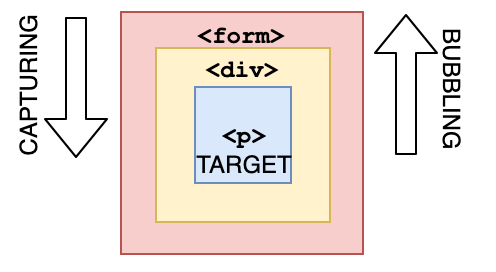###### 14. All object have prototypes.
• A: true
• B: false

Answer: B All objects have prototypes, except for the **base object**. The base object is the object created by the user, or an object that is created using the `new` keyword. The base object has access to some methods and properties, such as `.toString`. This is the reason why you can use built-in JavaScript methods! All of such methods are available on the prototype. Although JavaScript can't find it directly on your object, it goes down the prototype chain and finds it there, which makes it accessible for you.

###### 15. What's the output?
``````function sum(a, b) {
return a + b;
}

sum(1, '2');
``````
• A: `NaN`
• B: `TypeError`
• C: `"12"`
• D: `3`

Answer: C JavaScript is a **dynamically typed language**: we don't specify what types certain variables are. Values can automatically be converted into another type without you knowing, which is called _implicit type coercion_. **Coercion** is converting from one type into another. In this example, JavaScript converts the number `1` into a string, in order for the function to make sense and return a value. During the addition of a numeric type (`1`) and a string type (`'2'`), the number is treated as a string. We can concatenate strings like `"Hello" + "World"`, so what's happening here is `"1" + "2"` which returns `"12"`.

###### 16. What's the output?
``````let number = 0;
console.log(number++);
console.log(++number);
console.log(number);
``````
• A: `1` `1` `2`
• B: `1` `2` `2`
• C: `0` `2` `2`
• D: `0` `1` `2`

Answer: C The **postfix** unary operator `++`: 1. Returns the value (this returns `0`) 2. Increments the value (number is now `1`) The **prefix** unary operator `++`: 1. Increments the value (number is now `2`) 2. Returns the value (this returns `2`) This returns `0 2 2`.

###### 17. What's the output?
``````function getPersonInfo(one, two, three) {
console.log(one);
console.log(two);
console.log(three);
}

const person = 'Lydia';
const age = 21;

getPersonInfo`\${person} is \${age} years old`;
``````
• A: `"Lydia"` `21` `["", " is ", " years old"]`
• B: `["", " is ", " years old"]` `"Lydia"` `21`
• C: `"Lydia"` `["", " is ", " years old"]` `21`

Answer: B If you use tagged template literals, the value of the first argument is always an array of the string values. The remaining arguments get the values of the passed expressions!

###### 18. What's the output?
``````function checkAge(data) {
if (data === { age: 18 }) {
} else if (data == { age: 18 }) {
} else {
console.log(`Hmm.. You don't have an age I guess`);
}
}

checkAge({ age: 18 });
``````
• A: `You are an adult!`
• B: `You are still an adult.`
• C: `Hmm.. You don't have an age I guess`

Answer: C When testing equality, primitives are compared by their _value_, while objects are compared by their _reference_. JavaScript checks if the objects have a reference to the same location in memory.
The two objects that we are comparing don't have that: the object we passed as a parameter refers to a different location in memory than the object we used in order to check equality. This is why both `{ age: 18 } === { age: 18 }` and `{ age: 18 } == { age: 18 }` return `false`.

###### 19. What's the output?
``````function getAge(...args) {
console.log(typeof args);
}

getAge(21);
``````
• A: `"number"`
• B: `"array"`
• C: `"object"`
• D: `"NaN"`

Answer: C The rest parameter (`...args`) lets us "collect" all remaining arguments into an array. An array is an object, so `typeof args` returns `"object"`

###### 20. What's the output?
``````function getAge() {
'use strict';
age = 21;
console.log(age);
}

getAge();
``````
• A: `21`
• B: `undefined`
• C: `ReferenceError`
• D: `TypeError`

Answer: C With `"use strict"`, you can make sure that you don't accidentally declare global variables. We never declared the variable `age`, and since we use `"use strict"`, it will throw a reference error. If we didn't use `"use strict"`, it would have worked, since the property `age` would have gotten added to the global object.

###### 21. What's the value of `sum`?
``````const sum = eval('10*10+5');
``````
• A: `105`
• B: `"105"`
• C: `TypeError`
• D: `"10*10+5"`

Answer: A `eval` evaluates codes that's passed as a string. If it's an expression, like in this case, it evaluates the expression. The expression is `10 * 10 + 5`. This returns the number `105`.

###### 22. How long is cool_secret accessible?
``````sessionStorage.setItem('cool_secret', 123);
``````
• A: Forever, the data doesn't get lost.
• B: When the user closes the tab.
• C: When the user closes the entire browser, not only the tab.
• D: When the user shuts off their computer.

Answer: B The data stored in `sessionStorage` is removed after closing the _tab_. If you used `localStorage`, the data would've been there forever, unless for example `localStorage.clear()` is invoked.

###### 23. What's the output?
``````var num = 8;
var num = 10;

console.log(num);
``````
• A: `8`
• B: `10`
• C: `SyntaxError`
• D: `ReferenceError`

Answer: B With the `var` keyword, you can declare multiple variables with the same name. The variable will then hold the latest value. You cannot do this with `let` or `const` since they're block-scoped.

###### 24. What's the output?
``````const obj = { 1: 'a', 2: 'b', 3: 'c' };
const set = new Set([1, 2, 3, 4, 5]);

obj.hasOwnProperty('1');
obj.hasOwnProperty(1);
set.has('1');
set.has(1);
``````
• A: `false` `true` `false` `true`
• B: `false` `true` `true` `true`
• C: `true` `true` `false` `true`
• D: `true` `true` `true` `true`

Answer: C All object keys (excluding Symbols) are strings under the hood, even if you don't type it yourself as a string. This is why `obj.hasOwnProperty('1')` also returns true. It doesn't work that way for a set. There is no `'1'` in our set: `set.has('1')` returns `false`. It has the numeric type `1`, `set.has(1)` returns `true`.

###### 25. What's the output?
``````const obj = { a: 'one', b: 'two', a: 'three' };
console.log(obj);
``````
• A: `{ a: "one", b: "two" }`
• B: `{ b: "two", a: "three" }`
• C: `{ a: "three", b: "two" }`
• D: `SyntaxError`

Answer: C If you have two keys with the same name, the key will be replaced. It will still be in its first position, but with the last specified value.

###### 26. The JavaScript global execution context creates two things for you: the global object, and the "this" keyword.
• A: true
• B: false
• C: it depends

Answer: A The base execution context is the global execution context: it's what's accessible everywhere in your code.

###### 27. What's the output?
``````for (let i = 1; i < 5; i++) {
if (i === 3) continue;
console.log(i);
}
``````
• A: `1` `2`
• B: `1` `2` `3`
• C: `1` `2` `4`
• D: `1` `3` `4`

Answer: C The `continue` statement skips an iteration if a certain condition returns `true`.

###### 28. What's the output?
``````String.prototype.giveLydiaPizza = () => {
return 'Just give Lydia pizza already!';
};

const name = 'Lydia';

name.giveLydiaPizza();
``````
• A: `"Just give Lydia pizza already!"`
• B: `TypeError: not a function`
• C: `SyntaxError`
• D: `undefined`

Answer: A `String` is a built-in constructor, which we can add properties to. I just added a method to its prototype. Primitive strings are automatically converted into a string object, generated by the string prototype function. So, all strings (string objects) have access to that method!

###### 29. What's the output?
``````const a = {};
const b = { key: 'b' };
const c = { key: 'c' };

a[b] = 123;
a[c] = 456;

console.log(a[b]);
``````
• A: `123`
• B: `456`
• C: `undefined`
• D: `ReferenceError`

Answer: B Object keys are automatically converted into strings. We are trying to set an object as a key to object `a`, with the value of `123`. However, when we stringify an object, it becomes `"[object Object]"`. So what we are saying here, is that `a["[object Object]"] = 123`. Then, we can try to do the same again. `c` is another object that we are implicitly stringifying. So then, `a["[object Object]"] = 456`. Then, we log `a[b]`, which is actually `a["[object Object]"]`. We just set that to `456`, so it returns `456`.

###### 30. What's the output?
``````const foo = () => console.log('First');
const bar = () => setTimeout(() => console.log('Second'));
const baz = () => console.log('Third');

bar();
foo();
baz();
``````
• A: `First` `Second` `Third`
• B: `First` `Third` `Second`
• C: `Second` `First` `Third`
• D: `Second` `Third` `First`

Answer: B We have a `setTimeout` function and invoked it first. Yet, it was logged last. This is because in browsers, we don't just have the runtime engine, we also have something called a `WebAPI`. The `WebAPI` gives us the `setTimeout` function to start with, and for example the DOM. After the _callback_ is pushed to the WebAPI, the `setTimeout` function itself (but not the callback!) is popped off the stack.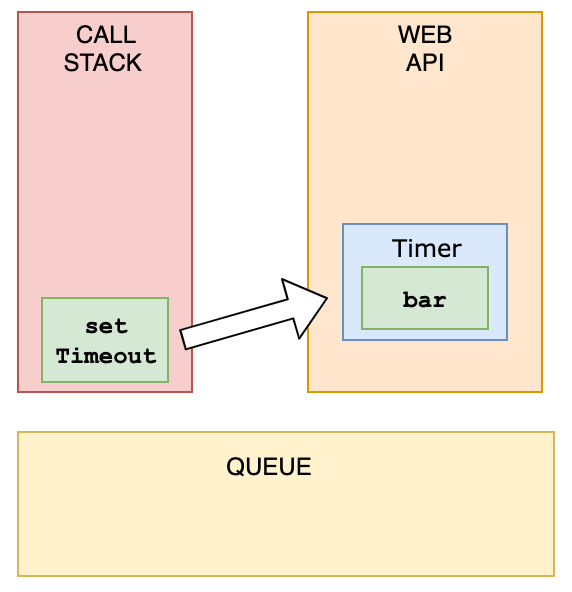Now, `foo` gets invoked, and `"First"` is being logged.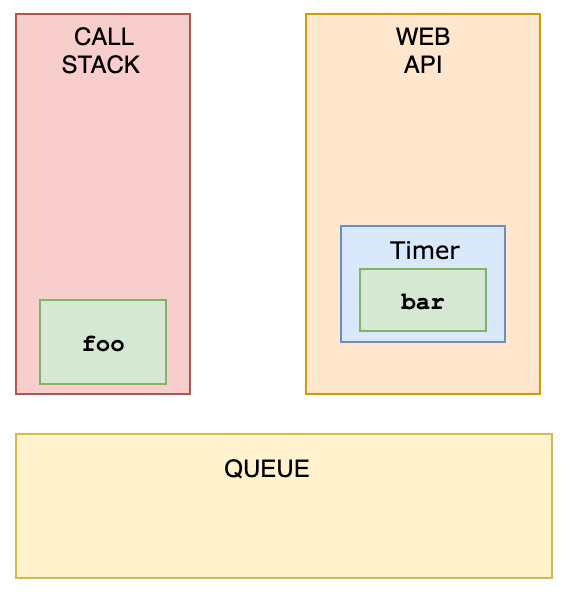`foo` is popped off the stack, and `baz` gets invoked. `"Third"` gets logged.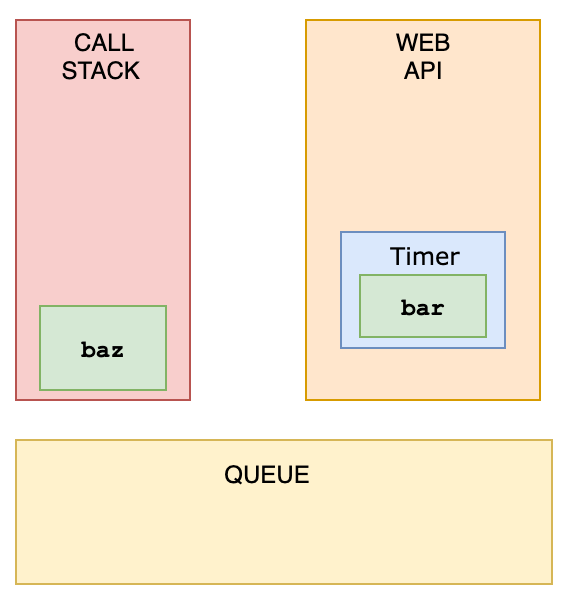The WebAPI can't just add stuff to the stack whenever it's ready. Instead, it pushes the callback function to something called the _queue_.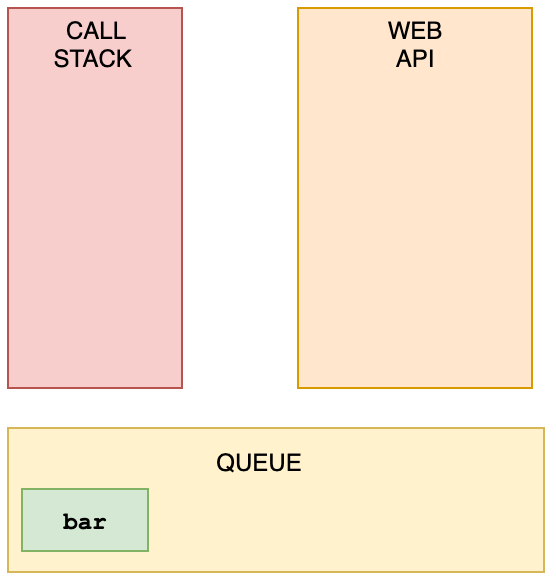This is where an event loop starts to work. An **event loop** looks at the stack and task queue. If the stack is empty, it takes the first thing on the queue and pushes it onto the stack.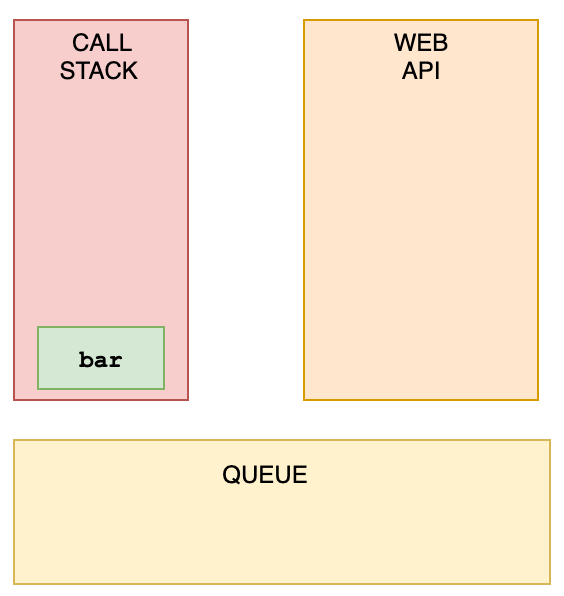`bar` gets invoked, `"Second"` gets logged, and it's popped off the stack.

###### 31. What is the event.target when clicking the button?
``````<div onclick="console.log('first div')">
<div onclick="console.log('second div')">
<button onclick="console.log('button')">
Click!
</button>
</div>
</div>
``````
• A: Outer `div`
• B: Inner `div`
• C: `button`
• D: An array of all nested elements.

Answer: C The deepest nested element that caused the event is the target of the event. You can stop bubbling by `event.stopPropagation`

###### 32. When you click the paragraph, what's the logged output?
``````<div onclick="console.log('div')">
<p onclick="console.log('p')">
</p>
</div>
``````
• A: `p` `div`
• B: `div` `p`
• C: `p`
• D: `div`

Answer: A If we click `p`, we see two logs: `p` and `div`. During event propagation, there are 3 phases: capturing, target, and bubbling. By default, event handlers are executed in the bubbling phase (unless you set `useCapture` to `true`). It goes from the deepest nested element outwards.

###### 33. What's the output?
``````const person = { name: 'Lydia' };

function sayHi(age) {
return `\${this.name} is \${age}`;
}

console.log(sayHi.call(person, 21));
console.log(sayHi.bind(person, 21));
``````
• A: `undefined is 21` `Lydia is 21`
• B: `function` `function`
• C: `Lydia is 21` `Lydia is 21`
• D: `Lydia is 21` `function`

Answer: D With both, we can pass the object to which we want the `this` keyword to refer to. However, `.call` is also _executed immediately_! `.bind.` returns a _copy_ of the function, but with a bound context! It is not executed immediately.

###### 34. What's the output?
``````function sayHi() {
return (() => 0)();
}

console.log(typeof sayHi());
``````
• A: `"object"`
• B: `"number"`
• C: `"function"`
• D: `"undefined"`

Answer: B The `sayHi` function returns the returned value of the immediately invoked function expression (IIFE). This function returned `0`, which is type `"number"`. FYI: there are only 7 built-in types: `null`, `undefined`, `boolean`, `number`, `string`, `object`, and `symbol`. `"function"` is not a type, since functions are objects, it's of type `"object"`.

###### 35. Which of these values are falsy?
``````0;
new Number(0);
('');
(' ');
new Boolean(false);
undefined;
``````
• A: `0`, `''`, `undefined`
• B: `0`, `new Number(0)`, `''`, `new Boolean(false)`, `undefined`
• C: `0`, `''`, `new Boolean(false)`, `undefined`
• D: All of them are falsy

Answer: A There are 8 falsy values: - `undefined` - `null` - `NaN` - `false` - `''` (empty string) - `0` - `-0` - `0n` (BigInt(0)) Function constructors, like `new Number` and `new Boolean` are truthy.

###### 36. What's the output?
``````console.log(typeof typeof 1);
``````
• A: `"number"`
• B: `"string"`
• C: `"object"`
• D: `"undefined"`

Answer: B `typeof 1` returns `"number"`. `typeof "number"` returns `"string"`

###### 37. What's the output?
``````const numbers = [1, 2, 3];
numbers = 11;
console.log(numbers);
``````
• A: `[1, 2, 3, 7 x null, 11]`
• B: `[1, 2, 3, 11]`
• C: `[1, 2, 3, 7 x empty, 11]`
• D: `SyntaxError`

Answer: C When you set a value to an element in an array that exceeds the length of the array, JavaScript creates something called "empty slots". These actually have the value of `undefined`, but you will see something like: `[1, 2, 3, 7 x empty, 11]` depending on where you run it (it's different for every browser, node, etc.)

###### 38. What's the output?
``````(() => {
let x, y;
try {
throw new Error();
} catch (x) {
(x = 1), (y = 2);
console.log(x);
}
console.log(x);
console.log(y);
})();
``````
• A: `1` `undefined` `2`
• B: `undefined` `undefined` `undefined`
• C: `1` `1` `2`
• D: `1` `undefined` `undefined`

Answer: A The `catch` block receives the argument `x`. This is not the same `x` as the variable when we pass arguments. This variable `x` is block-scoped. Later, we set this block-scoped variable equal to `1`, and set the value of the variable `y`. Now, we log the block-scoped variable `x`, which is equal to `1`. Outside of the `catch` block, `x` is still `undefined`, and `y` is `2`. When we want to `console.log(x)` outside of the `catch` block, it returns `undefined`, and `y` returns `2`.

###### 39. Everything in JavaScript is either a...
• A: primitive or object
• B: function or object
• C: trick question! only objects
• D: number or object

Answer: A JavaScript only has primitive types and objects. Primitive types are `boolean`, `null`, `undefined`, `bigint`, `number`, `string`, and `symbol`. What differentiates a primitive from an object is that primitives do not have any properties or methods; however, you'll note that `'foo'.toUpperCase()` evaluates to `'FOO'` and does not result in a `TypeError`. This is because when you try to access a property or method on a primitive like a string, JavaScript will implicitly wrap the primitive type using one of the wrapper classes, i.e. `String`, and then immediately discard the wrapper after the expression evaluates. All primitives except for `null` and `undefined` exhibit this behaviour.

###### 40. What's the output?
``````[[0, 1], [2, 3]].reduce(
(acc, cur) => {
return acc.concat(cur);
},
[1, 2],
);
``````
• A: `[0, 1, 2, 3, 1, 2]`
• B: `[6, 1, 2]`
• C: `[1, 2, 0, 1, 2, 3]`
• D: `[1, 2, 6]`

Answer: C `[1, 2]` is our initial value. This is the value we start with, and the value of the very first `acc`. During the first round, `acc` is `[1,2]`, and `cur` is `[0, 1]`. We concatenate them, which results in `[1, 2, 0, 1]`. Then, `[1, 2, 0, 1]` is `acc` and `[2, 3]` is `cur`. We concatenate them, and get `[1, 2, 0, 1, 2, 3]`

###### 41. What's the output?
``````!!null;
!!'';
!!1;
``````
• A: `false` `true` `false`
• B: `false` `false` `true`
• C: `false` `true` `true`
• D: `true` `true` `false`

Answer: B `null` is falsy. `!null` returns `true`. `!true` returns `false`. `""` is falsy. `!""` returns `true`. `!true` returns `false`. `1` is truthy. `!1` returns `false`. `!false` returns `true`.

###### 42. What does the `setInterval` method return in the browser?
``````setInterval(() => console.log('Hi'), 1000);
``````
• A: a unique id
• B: the amount of milliseconds specified
• C: the passed function
• D: `undefined`

Answer: A It returns a unique id. This id can be used to clear that interval with the `clearInterval()` function.

###### 43. What does this return?
``````[...'Lydia'];
``````
• A: `["L", "y", "d", "i", "a"]`
• B: `["Lydia"]`
• C: `[[], "Lydia"]`
• D: `[["L", "y", "d", "i", "a"]]`

Answer: A A string is an iterable. The spread operator maps every character of an iterable to one element.

###### 44. What's the output?
``````function* generator(i) {
yield i;
yield i * 2;
}

const gen = generator(10);

console.log(gen.next().value);
console.log(gen.next().value);
``````
• A: `[0, 10], [10, 20]`
• B: `20, 20`
• C: `10, 20`
• D: `0, 10 and 10, 20`

Answer: C Regular functions cannot be stopped mid-way after invocation. However, a generator function can be "stopped" midway, and later continue from where it stopped. Every time a generator function encounters a `yield` keyword, the function yields the value specified after it. Note that the generator function in that case doesn’t _return_ the value, it _yields_ the value. First, we initialize the generator function with `i` equal to `10`. We invoke the generator function using the `next()` method. The first time we invoke the generator function, `i` is equal to `10`. It encounters the first `yield` keyword: it yields the value of `i`. The generator is now "paused", and `10` gets logged. Then, we invoke the function again with the `next()` method. It starts to continue where it stopped previously, still with `i` equal to `10`. Now, it encounters the next `yield` keyword, and yields `i * 2`. `i` is equal to `10`, so it returns `10 * 2`, which is `20`. This results in `10, 20`.

###### 45. What does this return?
``````const firstPromise = new Promise((res, rej) => {
setTimeout(res, 500, 'one');
});

const secondPromise = new Promise((res, rej) => {
setTimeout(res, 100, 'two');
});

Promise.race([firstPromise, secondPromise]).then(res => console.log(res));
``````
• A: `"one"`
• B: `"two"`
• C: `"two" "one"`
• D: `"one" "two"`

Answer: B When we pass multiple promises to the `Promise.race` method, it resolves/rejects the _first_ promise that resolves/rejects. To the `setTimeout` method, we pass a timer: 500ms for the first promise (`firstPromise`), and 100ms for the second promise (`secondPromise`). This means that the `secondPromise` resolves first with the value of `'two'`. `res` now holds the value of `'two'`, which gets logged.

###### 46. What's the output?
``````let person = { name: 'Lydia' };
const members = [person];
person = null;

console.log(members);
``````
• A: `null`
• B: `[null]`
• C: `[{}]`
• D: `[{ name: "Lydia" }]`

Answer: D First, we declare a variable `person` with the value of an object that has a `name` property.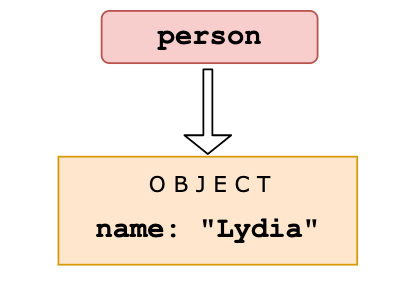Then, we declare a variable called `members`. We set the first element of that array equal to the value of the `person` variable. Objects interact by _reference_ when setting them equal to each other. When you assign a reference from one variable to another, you make a _copy_ of that reference. (note that they don't have the _same_ reference!)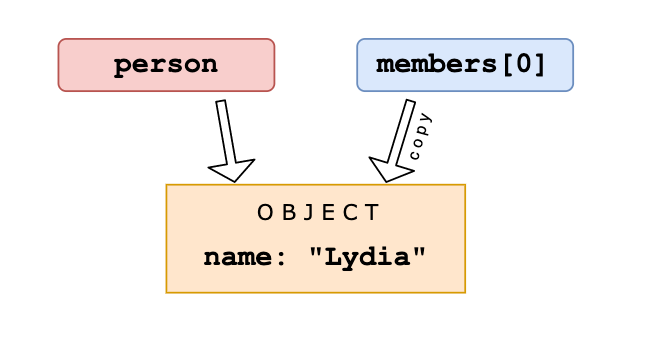Then, we set the variable `person` equal to `null`.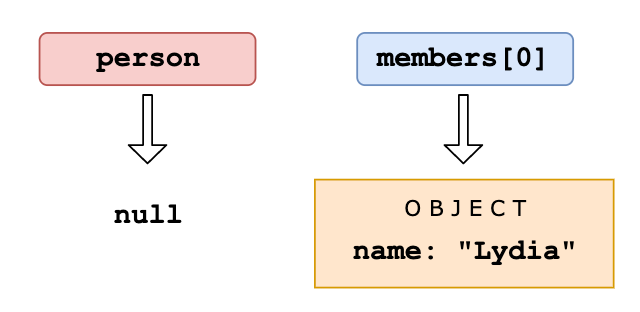We are only modifying the value of the `person` variable, and not the first element in the array, since that element has a different (copied) reference to the object. The first element in `members` still holds its reference to the original object. When we log the `members` array, the first element still holds the value of the object, which gets logged.

###### 47. What's the output?
``````const person = {
name: 'Lydia',
age: 21,
};

for (const item in person) {
console.log(item);
}
``````
• A: `{ name: "Lydia" }, { age: 21 }`
• B: `"name", "age"`
• C: `"Lydia", 21`
• D: `["name", "Lydia"], ["age", 21]`

Answer: B With a `for-in` loop, we can iterate through object keys, in this case `name` and `age`. Under the hood, object keys are strings (if they're not a Symbol). On every loop, we set the value of `item` equal to the current key it’s iterating over. First, `item` is equal to `name`, and gets logged. Then, `item` is equal to `age`, which gets logged.

###### 48. What's the output?
``````console.log(3 + 4 + '5');
``````
• A: `"345"`
• B: `"75"`
• C: `12`
• D: `"12"`

Answer: B Operator associativity is the order in which the compiler evaluates the expressions, either left-to-right or right-to-left. This only happens if all operators have the _same_ precedence. We only have one type of operator: `+`. For addition, the associativity is left-to-right. `3 + 4` gets evaluated first. This results in the number `7`. `7 + '5'` results in `"75"` because of coercion. JavaScript converts the number `7` into a string, see question 15. We can concatenate two strings using the `+`operator. `"7" + "5"` results in `"75"`.

###### 49. What's the value of `num`?
``````const num = parseInt('7*6', 10);
``````
• A: `42`
• B: `"42"`
• C: `7`
• D: `NaN`

Answer: C Only the first numbers in the string is returned. Based on the _radix_ (the second argument in order to specify what type of number we want to parse it to: base 10, hexadecimal, octal, binary, etc.), the `parseInt` checks whether the characters in the string are valid. Once it encounters a character that isn't a valid number in the radix, it stops parsing and ignores the following characters. `*` is not a valid number. It only parses `"7"` into the decimal `7`. `num` now holds the value of `7`.

###### 50. What's the output?
``````[1, 2, 3].map(num => {
if (typeof num === 'number') return;
return num * 2;
});
``````
• A: `[]`
• B: `[null, null, null]`
• C: `[undefined, undefined, undefined]`
• D: `[ 3 x empty ]`